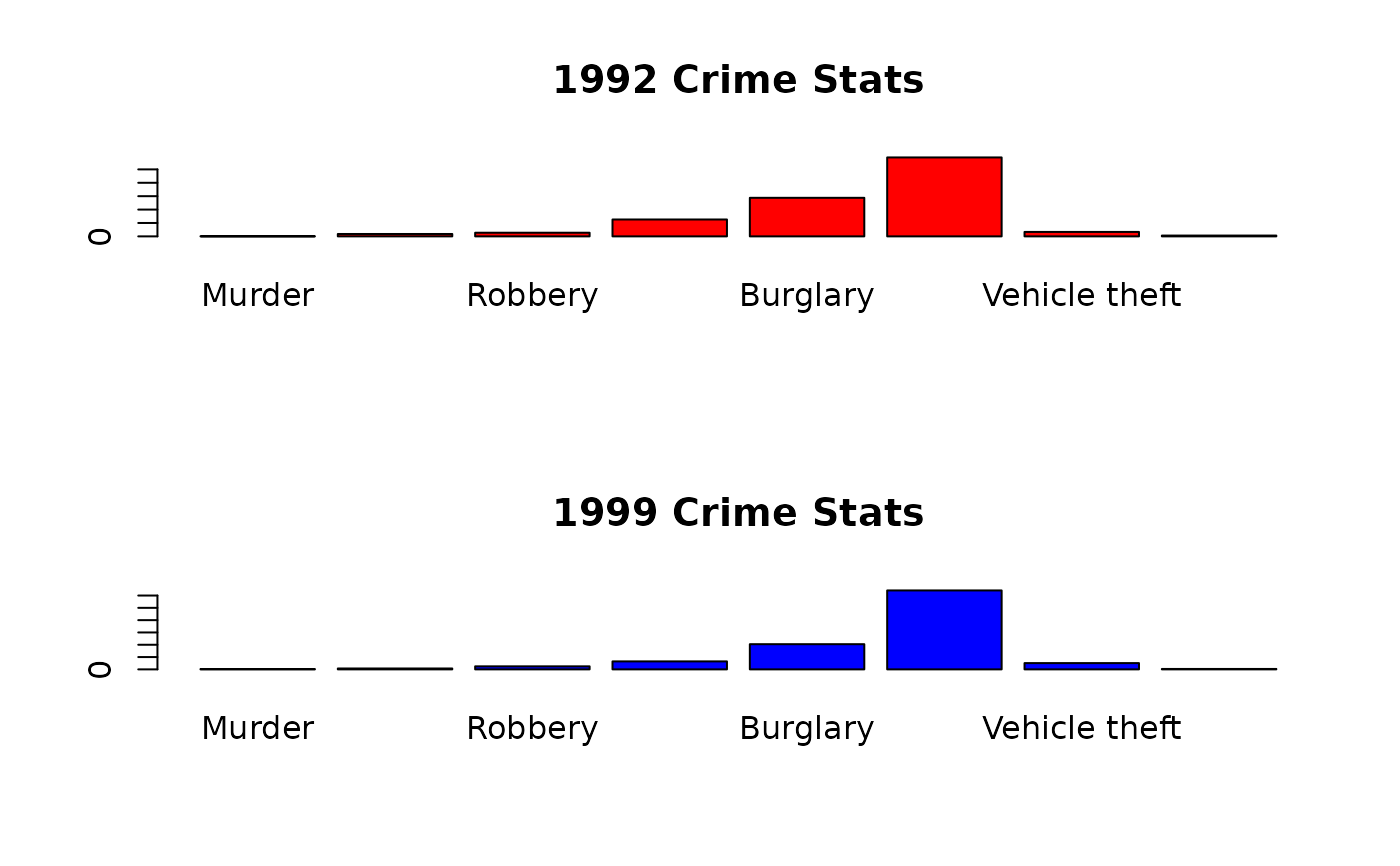Data used in Exercise 1.23 and 2.79

Abilene

## Format

A data frame/tibble with 16 observations on three variables

crimetype

a character variable with values Aggravated assault, Arson, Burglary, Forcible rape, Larceny theft, Murder, Robbery, and Vehicle theft.

year

a factor with levels 1992 and 1999

number

number of reported crimes

## Source

Uniform Crime Reports, US Dept. of Justice.

## Examples


par(mfrow = c(2, 1))
barplot(Abilene$number[Abilene$year=="1992"],
names.arg = Abilene$crimetype[Abilene$year == "1992"],
main = "1992 Crime Stats", col = "red")
barplot(Abilene$number[Abilene$year=="1999"],
names.arg = Abilene$crimetype[Abilene$year == "1999"],
main = "1999 Crime Stats", col = "blue")par(mfrow = c(1, 1))

if (FALSE) {
library(ggplot2)
ggplot2::ggplot(data = Abilene, aes(x = crimetype, y = number, fill = year)) +
geom_bar(stat = "identity", position = "dodge") +
theme_bw() +
theme(axis.text.x = element_text(angle = 30, hjust = 1))
}